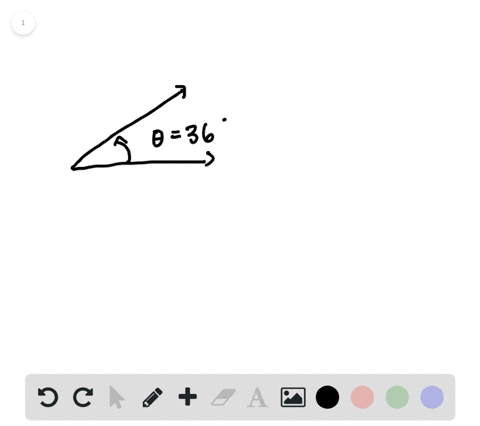🎉 The Study-to-Win Winning Ticket number has been announced! Go to your Tickets dashboard to see if you won! 🎉View Winning Ticket### Coterminal Angles in Degrees In Exercises 5 and 6…

00:50University of Southern California
Problem 4

# Characteristics of a Graph In your own words, describe the meaning of amplitude and period.

## Discussion

You must be signed in to discuss.

## Video Transcript

we're asked to describe the meaning of the two words, amplitude and period in our own words. So in the context of trigonometry, HQ functions and graphing, these are two very important characteristic terms. Now the 1st 1 I'm going to describe is amplitude amplitude, and how I like to think about the amplitude of a function is simply the distance or half the distance between the highest and lowest point on our periodic graph. So if you look here, well, look here, this topless peak in the bottom of this trough, and we find that distance between them. Okay, we'll call it some height, age, and the amplitude is simply that height age divided by two. So I'll just put equals H divided by two. Another way to think about it is it's that distance of oscillation from the center of our graph, so you can see how our graph oscillates about this center line R X axis. In this case, the distance from the highest point in the lowest point is the same one either side, and that's the amplitude now moving on to our concept of what a period is. I like to think of the period as the distance. Our graph travels in one full revolution of the units circle. So when you think about our periodic functions there called pure attic functions because they repeat and if you look here, you see, we have to fall repetitions one into now, the period of a function is simply the length of one of those repetitions.